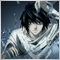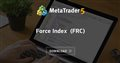# Combine force index indicator with moving average "use on Previous Indicator's data"77

There is an option for Moving averages named "use on: Previous Indicator's data ".

So if we have an indicator which current value is about 1000, but the value of ma indicator is 1.229, the ma value get an offset to be around 1000. (i dont exactly know how this works)

This works perfectly on mt5 in an indicator window like adding force index, put ma with previuous indicators data.

But for my EA i dont have this option and i need it to recieve crosses.

How i can realize this setup as a standalone indicator.

I tried something like that, but im not able to get the second buffer working:

```#include <MovingAverages.mqh>

int OnCalculate(const int rates_total,
const int prev_calculated,
const datetime &time[],
const double &open[],
const double &high[],
const double &low[],
const double &close[],
const long &tick_volume[],
const long &volume[],
{

for(int i2=inpPeriod;i2<rates_total && !IsStopped();i2++){

double FIArray[];
ArraySetAsSeries( FIArray , true);
int FIDefinition=iForce(_Symbol,_Period, inpPeriod, MODE_SMA, TICK_VOLUME );
CopyBuffer( FIDefinition ,0,rates_total-i2-1,1, FIArray);

FIBuffer[i2] = FIArray  ;

double MAArray[];
ArraySetAsSeries(MAArray, true);
int MADefinition= SimpleMAOnBuffer(rates_total,prev_calculated,rates_total-i2+inpPeriod-1,inpPeriod, FIBuffer , MAArray);

MaBuffer[i2] = MAArray;

}

return(rates_total);
}```Force Index (FRC)
• www.mql5.com
The Force Index Indicator was developed by Alexander Elder. This index measures the Bulls Power at each increase, and the Bulls Power at each decrease.